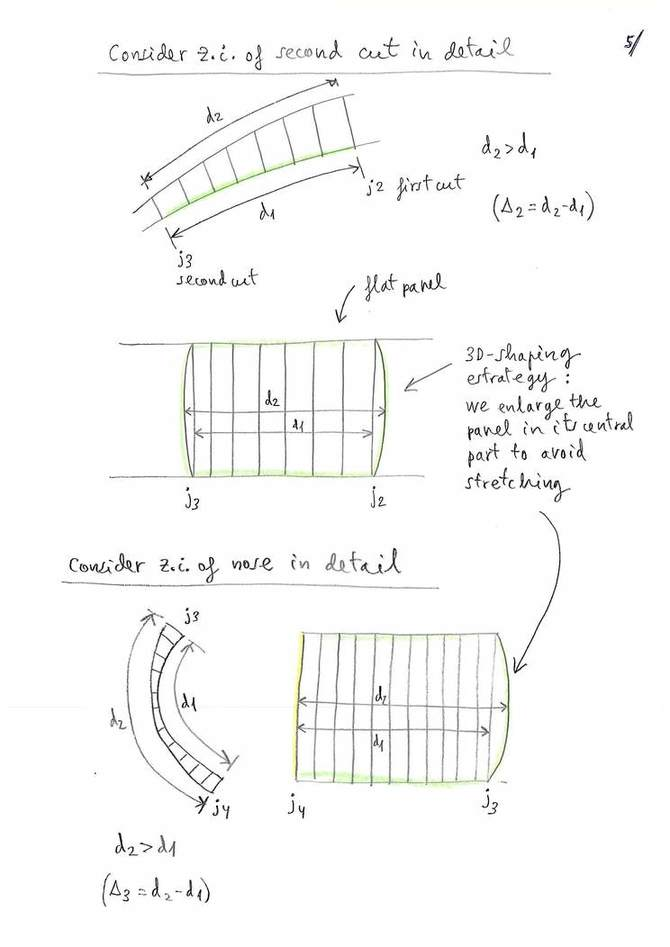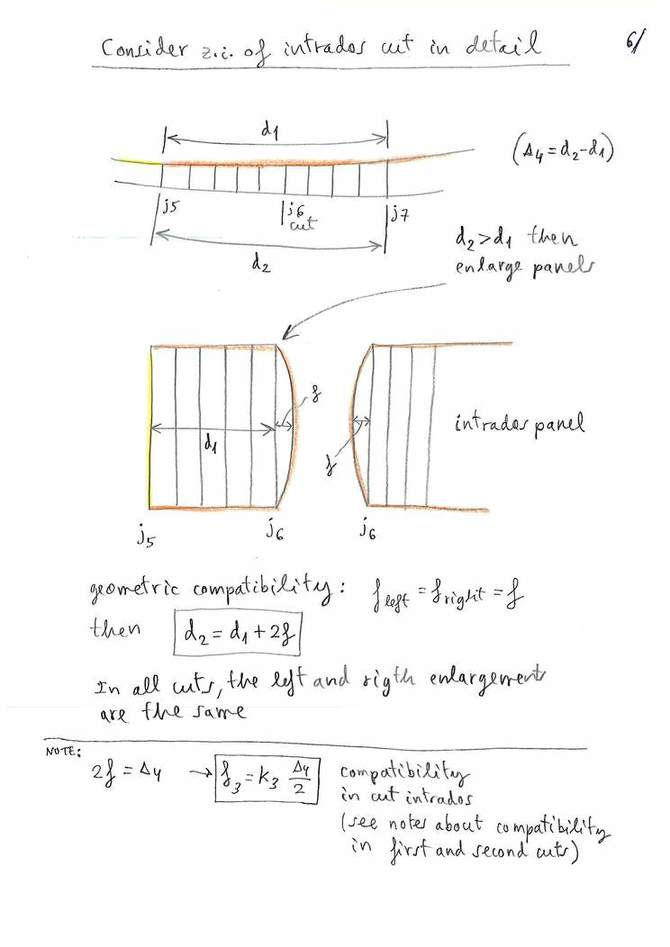3D-SHAPING

PART 2: 3D-SHAPING PROGRAMMING ESTRATEGY

.
PART 2: 3D-SHAPING PROGRAMMING ESTRATEGYPART 4: A device for experimental study of deformations and wrinkles

In this PART 2 we continue studying the concept of 3D-shaping, started
in part 1.

This document shows the details and the strategy used in the programming of the module 3D-shaping of Laboratori d'envol. We come back to review the basics, and we propose one method in particular. Now, we just have to move these ideas to a few lines of Fortran code, and integrate the code within the next version of the program LEparagliding. The work is simple. Only it is necessary to define correctly the data, and then the logic becomes trivial. Only the part corresponding to drawing the different parts separated, sorted and marked, will require a detailed and hard work.

To use the program LEparagliding is not necessary to study all these ideas... (!), these are directed only to the researchers of the details and the concepts used inside the program. To use the program you only need to know the meaning of some parameters that we will explain latter in the manual.

The drawings are self explanatory (this is the intention!):Figure 1Figure 2Figure 3Figure 4Figure 5Figure 6Figure 7Figure 8. See note ** belowFigure 9. See note ** below

(**) Note: These examples of data are a version simplied. In the final version we have added the concept of "groups" to define different values for groups of profiles. Also added a few print settings of the results to the files to dxf, and the possibility of generating other files as well as .stl. View the last version of the LEparagliding manual, section 29.Figure 10

Let's see in detail how to do calculation of the arches of the cuts (step 4 refered in figure 10):

Briefly, the system of 3D-shaping "type 1" proposed by the Laboratori consist of making one or more transverse cuts to the panels, and add the amount of fabric necessary to cover the "cracks" that appear on the surfaces due to the higher thickness of the median ovalized profiles and the pronounced curvature in the nose area.

The cracks are covered by adding arcs of fabric to the straight edges. The arches to the right and left of the cut must be of equal length in order to sew with a perfect match.

The arches are defined by the length of the center arrow (f).

This arrow is calculated automatically by the program, taking into account the increases in length in the areas to the right and to the left of the cut point. In addition, the depth of the arrow is adjustable by the designer with a coefficient around the value 1.0. The value 0.0 means not to apply 3D effect, the value 1.0 means to consider the values calculated automatically, and other lower values (0.8) or higher (1.1) reduce or amplify the 3D effect.

Consider all the points defining the profile and the following control points in te nose area (exactly as show in figure 3 above):
j1: start reference point
j4: in vent
j5: out vent
j7: end reference point

Consider the following "zones":
zone 1: between point j1 and j2
zone 2: between point j2 and j3
zone 3: between point j3 and j4
zone 4: between point j4 and j5
zone 5: between point j5 and j6
zone 6: between point j6 and j7

In each zone 1,2,3,4,5,6 (portion of panel) consider the following parameters:
d1: Length of the segment of the median airfoil without ovalization
d2:
Length of the segment of the median airfoil with ovalization
d2-d1: Difference of lengths
d3: Increase of the panel at the initial edge
d4: Increase of the panel at the edge end

Now let's calculate the arrow length needed for each cut
(see figures below):

- Case 0 cuts in extrados: do nothing!  :)
- Case 1 cut in extrados:

We consider zone 1 beetwee point j1 and j2, and "extended zone 3" between point  j2 and j4.
Let's define the arrow in cut 1 as:

f13=0.5x(d4(1)+d3(3))*k1

Where k1 is the depth coefficient around 1.0

- Case 2 cuts in extrados:

Let's define the arrow in cut 1 as: f12=0.5x(d4(1)+d3(2))*k1
Let's define the arrow in cut 2 as: f23=0.5x(d4(2)+d3(3))*k2

Where k1, k2 are the depth coefficients.

-
Case 0 cuts in intrados: do nothing!  :)
- Case 1 cut in intrados:

We consider zone 5 beetwee point j5 and j6, and zone 6 between point  j6 and j7.
Let's define the arrow in cut as:

f56=0.5x(d4(5)+d3(6))*k3

Where k3 is the depth coefficient around 1.0Figure 11Figure 12

For each median airfoil between rib i and tip rib, program LEparagliding computes and prints the numerical results (d1,d2,d2-d1,f) in tabular form in the section 9 of file lep-out.txt

The next step is the drawing the arcs at the edges of the cuts...:Figure 13. (Explanation soon...)

In relation to the step 7 programming, this is optional. But we can imagine a .DXF/.STL tessellation file that can be visualized with OpenSCAD or FreeCAD and will be very interesting... Crazy Idea, I imagine as possible. And yes...:Figure 14

This page shows a simple method to define a 3D tessellation for the ovalized surfaces. Once calculated the arc that joins the points to the right and left of two contiguous profiles, we only need to split this arc into smaller sections, for example 8. It is necessary to define a local system of coordinates in the own plane of the arc, to make the division in a simple form (for example, calculating the coordinates relative to the center of the arc). And apply the formulas of transformation of coordinates from local to global by translation and rotation:Figure 15

Now only it is necessary to convert these ideas in Fortran... :)LEP-3.03 testing, starting code for 3D-shaping...2D intermediate airfoils (white) 2D ovalized airfoils (cyan)2D intermediate airfoils (white) 2D ovalized airfoils (cyan)

Module 3D-shaping for LEparagliding will be programmed in February-March 2020 according to the plans. This plans will be possible thanks to the support of Scott Roberts from USA (Fluid Wings https://www.fluidwings.com/ ).